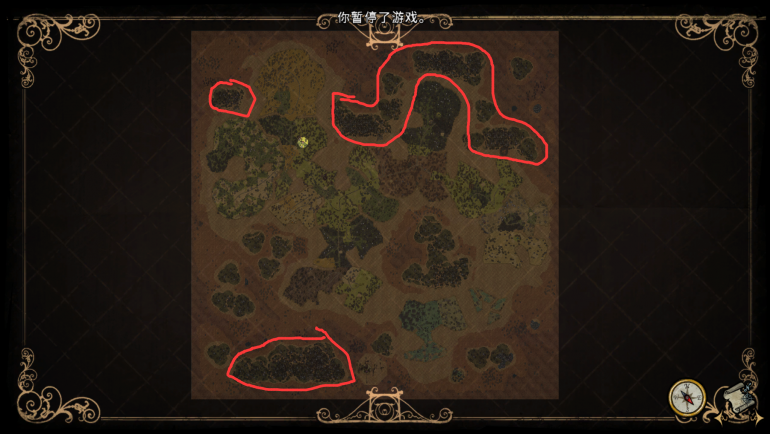# A big possible flaw in ocean terrain generation code

## Recommended Posts

I increased the number of waterlogs in a map by 30 times and allowed stacking to calculate the distribution pattern of waterlogs. Afterwards, I discovered a possible flaw in terrain generation.

You can see that the water wood is mainly concentrated in these three linear red box areas, and in the dozens of maps I generated, the water wood is basically generated in this form as a linear area.

This is because when the initial generation point of waterlog cannot meet the conditions for water wood generation, the water wood scene will search for the next judgment point in the positive direction of the x-axis. The initial generation point of water wood is random across the entire map, which means that when the initial generation point is randomly located on the mainland, the final generation position is often the seaside in the positive x-axis direction of the mainland. If the sea area in the positive x-axis direction does not have enough generating space, then water trees are more likely to generate on the map edge in the negative x-axis direction. (scripts/map/ocean_gen.lua: 766-813 function findLayoutPositions)

local function findLayoutPositions(size, edge_dist, populating_tile, count, min_dist_from_land)
local positions = {}
edge_dist = edge_dist or 0
min_dist_from_land = min_dist_from_land or 0

local width, height = world:GetWorldSize()
local adj_width, adj_height = width - 2 * edge_dist - size, height - 2 * edge_dist - size
local i, j = 0, 0

while j < adj_height and (count == nil or #positions < count) do
local y = ((start_y + j) % adj_height) + edge_dist
while i < adj_width and (count == nil or #positions < count) do
-- check the corners first
local x = ((start_x + i) % adj_width) + edge_dist
local x2, y2 = x + size - 1, y + size - 1
if checkTile(x2 + min_dist_from_land, y - min_dist_from_land, populating_tile) and checkTile(x2 + min_dist_from_land, y2 + min_dist_from_land, populating_tile) then
if checkTile(x - min_dist_from_land, y - min_dist_from_land, populating_tile) and checkTile(x - min_dist_from_land, y2 + min_dist_from_land, populating_tile) then
--print("Found 4 corners", x, y, x2, y2)
--check all tiles
local ok, last_x, last_y = checkAllTiles(populating_tile, x - min_dist_from_land, y - min_dist_from_land, x2 + min_dist_from_land, y2 + min_dist_from_land)
if ok == true then
--bottom-left
--print(string.format("Location found (%4.2f, %4.2f)", x, y))
--local adj = 0.5 * (size - actualsize)
--return {x + adj, y2 - adj} --{0.5 * (x + x2), 0.5 * (y + y2)}
table.insert(positions, {x = x, y = y, x2 = x2, y2 = y2, size = size})
i = i + size + 1
else
--print(string.format("Failed at (%4.2f, %4.2f) skip, (%4.2f, %4.2f)", last_x, last_y, x, y))
last_x = math.clamp(last_x, x, x2)
i = i + last_x - x + 1
end
else
i = i + 1
end
else
--print(string.format("Failed on x2, skip (%4.2f, %4.2f)", x, y))
i = i + size + 1
end
end
j = j + 1
i = 0
end

return positions
end

This code is used to generate water logs and salt mines. I guess the purpose of writing this is to allow most of the waterlogs and salt mines to be generated closer to the mainland, but the final result is that the number of water logs and salt mines in the positive x-axis direction is much higher than in other directions of the sea. My suggestion is to randomize the x-direction and y-direction of this algorithm, so that the number of waterlog and salt mines in the water distributed in the four directions of the sea area will be relatively average and reasonable.

This shows a bad situation where most of the underwater trees are distributed along the coast in the positive direction of the x-axis, while when there is not enough generating space, a large number of waterlog are distributed in the negative direction of the x-axis.This post was generated using translation software, and any issues can be pointed out. Thank you for your patient reading!